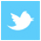A Crowd-sourced Cookbook on Writing Great Android® Apps### Using AndroidPlot to Display Charts and Graphs

In Chapter: Graphics
Author: Rachee Singh ('racheesingh')
Published? true
FormatLanguage: WikiFormat

### Problem:

Depicting data graphically in an Android application.

### Solution:

There are many 3rd party graph libraries for Android available. In this example we make use of AndroidPlot library (open source) to depict a simple graph.

### Discussion:

Step 1: Download AndroidPlot library from here: http://androidplot.com/wiki/Download (any version).

Step 2: Create a new Android project and add the AndroidPlot library to the new project. To do this, create a new folder in the project folder by the name 'lib'. To this folder add the downloaded AndroidPlot jar file (named something like this: 'Androidplot-core-0.4a-release.jar'). (At this stage you should be having directories like src, res, gen, lib.)

Step 3: To use the library, it must be added to the build path. For this, in Eclipse, right click the .jar you added and select the "Build Path -- Add to Build Path" option. This will show another directory called 'Referenced Libraries' in the Eclipse project.

Step 4: In our sample application, we are hard-coding some data and showing the plot corresponding to the data in the application. So, we require to add an XY plot to our XML layout (main.xml). Here's what main.xml looks like with a XYPlot component in a linear layout:

```<?xml version="1.0" encoding="utf-8"?>
<LinearLayout xmlns:android="http://schemas.android.com/apk/res/android"
android:orientation="vertical"
android:layout_width="fill_parent"
android:layout_height="fill_parent"
>
<com.androidplot.xy.XYPlot
android:id="@+id/mySimpleXYPlot"
android:layout_width="fill_parent"
android:layout_height="wrap_content"
title="Stats"/>
</LinearLayout>
```

Step 5: Get a reference to the XYplot defined in the XML.

```<source lang='java'>
mySimpleXYPlot = (XYPlot) findViewById(R.id.mySimpleXYPlot);
</source>
```

Step 6: Initialize two arrays of numbers for which the plot will be displayed.

```<source lang='java'>
// Create two arrays of y-values to plot:
Number[] series1Numbers = {1, 8, 5, 2, 7, 4};
Number[] series2Numbers = {4, 6, 3, 8, 2, 10};
</source>
```

Step 7: Turn the above arrays into XYSeries:

```<source lang='java'>
XYSeries series1 = new SimpleXYSeries(
Arrays.asList(series1Numbers),          // SimpleXYSeries takes a List so turn our array into a List
SimpleXYSeries.ArrayFormat.Y_VALS_ONLY, // Y_VALS_ONLY means use the element index as the x value
"Series1");                             // Set the display title of the series
XYSeries series2 = new SimpleXYSeries(Arrays.asList(series2Numbers), SimpleXYSeries.ArrayFormat.Y_VALS_ONLY,
"Series2");
</source>
```

Step 8: Create a formatter to use for drawing a series using LineAndPointRenderer:

```<source lang='java'>
LineAndPointFormatter series1Format = new LineAndPointFormatter(
Color.rgb(0, 200, 0),                   // line color
Color.rgb(0, 100, 0),                   // point color
Color.rgb(150, 190, 150));              // fill color (optional)
</source>
```

Step 9: Add series1 and series2 to the xyplot:

```<source lang='java'>
mySimpleXYPlot.addSeries(series1, series1Format);
mySimpleXYPlot.addSeries(series2, new LineAndPointFormatter(Color.rgb(0, 0, 200), Color.rgb(0, 0, 100), Color.rgb(150, 150, 190)));
</source>
```

Step 10: Make it look cleaner:

```<source lang='java'>
// Reduce the number of range labels
mySimpleXYPlot.setTicksPerRangeLabel(3);

// By default, AndroidPlot displays developer guides to aid in laying out your plot.
// To get rid of them call disableAllMarkup():
mySimpleXYPlot.disableAllMarkup();

mySimpleXYPlot.getBackgroundPaint().setAlpha(0);
mySimpleXYPlot.getGraphWidget().getBackgroundPaint().setAlpha(0);
mySimpleXYPlot.getGraphWidget().getGridBackgroundPaint().setAlpha(0);
</source>
```

Step 11: Run the application! This is how it looks:### Download:

The source code for this project can be downloaded from https://docs.google.com/leaf?id=0B_rESQKgad5LNTJjMDQ2MTktZjAzMi00ZjBkLWFhOTktZjA5OWY4YjE2MTRh&hl=en_US .

### Download:

The source code for this project is in the Android Cookbook repository, http://github.com/IanDarwin/Android-Cookbook-Examples/tree/master/AndroidPlot.